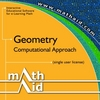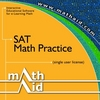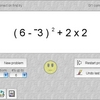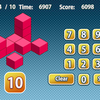# Mathaid algebra ii

Highly interactive tutorials and self-test system for individual e-learning, home schooling, college and high school computer learning centers, and distance learning. The product emphasizes on building problem-solving skills. Tutorials include the reviews of basic concepts, interactive examples, and standard problems with randomly generated parameters. The self-test system allows selecting topics and length for a test, saving test results, and getting the test review. Topics covered: rectangular coordinate system, functions and graphs, linear equations and inequalities in one variable, systems of linear equations and inequalities, determinants and Cramer's rule, operations with polynomials, factoring polynomials, roots of polynomial equations, rational expressions, exponents and radicals, complex numbers, quadratic functions, conic sections, exponential and logarithmic functions, sequences and series, binomial theorem, counting principles. The demo version contains selected lessons fro

## Related software (5)## MathAid Geometry

Tutorial contains basic concepts, interactive examples, and problems with randomly generated parameters. A customer is allowed to select chapters for a test, get the test review, ...## MathAid SAT. Math Practice

Tutorial contains basic concepts, interactive examples, and problems with randomly generated parameters. A customer is allowed to select chapters for a test, get the test review, ...## Smooth Operators

Smooth Operators is a complete solution for learning, practicing, and testing the order of operations. An interactive lesson teaches concepts. Practice by clicking operands, or tes ...## Count the Cubes

Can you spot the exact number of cubes immediately?## Geometry Quiz

Challenge your mind and try to solve all equations.